# Unijunction Transistor (UJT)

## Unijunction Transistor (UJT)

A unijunction transistor  (UJT) is a three terminal semiconductor switching device.

This device has a unique characteristics that when it is triggered, the emitter current increases regeneratively until it is imited by emitter power supply.

Due to this characteristics, the unijunction transistor can be employed in a variety of applications e.g., switching, pulse generator, saw-tooth generator etc.

### Construction of UJT

Fig.1 shows the basic structure of a unijunction transistor.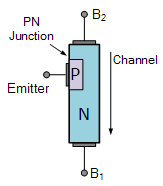Fig.1

It consists of an n-type silicon bar with an electrical connection on each end.

The leads to those connections are called base leads base-one B1 and base two B2.

Part way along the bar between the two bases, nearer to Bthan B1.a pn junction is formed between a p-type emitter and the bar. The lead to this junction is called the emitter lead E.

Fig.2. shows the symbol of unijunction transistor. Note that emitter is shown closer to Bthan B1.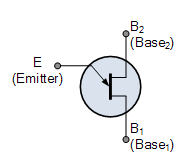Fig.2

The following points may be noted about the UJT:

1. Since the device has one pn junction and three leads, it is commonly called a unijunction transistor.
2. With only one pn junction, the device is really a form of diode. Because the two base terminals are taken from one section of the diode, this device is also called double-based diode.
3. The emitter is heavily doped having many holes. The n-region, however, is lightly doped. For this reason, the resistance between the base terminals is very high when emitter lead is open.

### Operation of UJT

Fig.3  shows the basic circuit operation of a unijunction transistor.

Fig.3(i)                                                                                                    Fig.3 (ii)

The device has normally Bpositive w.r.t. B1.

(i) If voltage VBB is applied between Band B1with emitter open, a voltage gradient is established along the n-type bar.

Since the emitter is located nearer to B2, more than half of  VBB appears between the emitter and B1.

The voltage Vbetween emitter and Bestablishes a reverse bias on the pn junction and the emitter current is cut off.

Of course, a small leakage current flows from Bto emitter due to minority carriers.

(ii) If a positive voltage is applied at the emitter, the pn junction will remain reverse biased so long as the input voltage is less than V1.

If the input voltage to the emitter exceeds V1, the pn junction becomes forward biased.

Under these conditions, holes are injected from p-type material into the n-type bar.

These holes are repelled by positive B2 terminal and they are attracted towards Bterminal of the bar.

This accumulation of the holes in the emitter to Bregion results in the decrease of resistance in this section of the bar.

The result is that internal voltage drop from emitter to Bis decreased and hence the emitter current Iincreases.

As more holes are injected, a condition of saturation will eventually be reached.

At this point, the emitter current is limited by emitter power supply only. The device is now in the ON sate.

(iii) If a negative pulse is applied to the emitter, the pn junction is reverse biased and the emitter current is cut off. The device is then said to be in the OFF state.

### Equivalent Circuit of a UJT

Fig.4 shows the equivalent circuit of a UJT.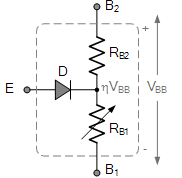Fig.4

The resistance of the silicon bar is called the inter-base resistance RBB.

The inter-base resistance is represented by two resistors in series such as:

(i) RB2 is the resistance of silicon bar between Band the point at which the emitter junction lies.

(ii) RB1 is the resistance of the bar between Band emitter junction. This resistance is shown variable because its value depends upon the bias voltage across the pn junction.

The pn junction is represented in the emitter by a diode D.

The circuit action of a UJT can be explained more clearly from its equivalent circuit.

(i)  With no voltage applied to the UJT, the inter-base resistance is given by ;

RBB = RB1 + RB2

(ii) If a voltage VBB is applied between the bases with emitter open,  the voltage will divide up across RB1 and RB2.

Voltage across RB1,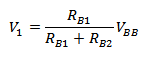Or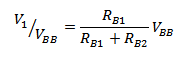The ratio V1/ VBB is called intrinsic stand-off ratio and is represented by η.

Hence,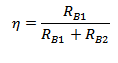The value of η lies between 0.51 and 0.82.

So voltage across RB1  = ηVBB

The voltage ηVBB appearing across RB1 reverse biases the diode. Therefore, the emitter current is zero.

(iii) If now a progressively rising voltage is applied to the emitter, the diode will become forward biased when input voltage exceeds ηVBB by VD, the forward voltage drop across the silicon diode i.e.

Where V= Peak point voltage

V= forward voltage drop across silicon diode (≈0.7 V)

When the diode D starts conducting, holes are injected from p-type material to the n-type bar. These holes are swept down towards the terminal B1. This decreases the resistance between emitter and B1( indicated by variable resistance RB1) and hence the internal drop from emitter to B1. The emitter current now increases regeneratively until it is limited by the emitter power supply.

##### Conclusion

When the input positive voltage  to the emitter is less than peak-point voltage VP, the pn junction remains reverse biased and the emitter current is practically zero.

However, when the input voltage exceeds VP, RB1 falls from several thousand ohms to a small value. The diode is now forward biased and the emitter current quickly reaches to a saturation value limited by RB1 and forward resistance of pn-junction.

You Might Like The Following Articles